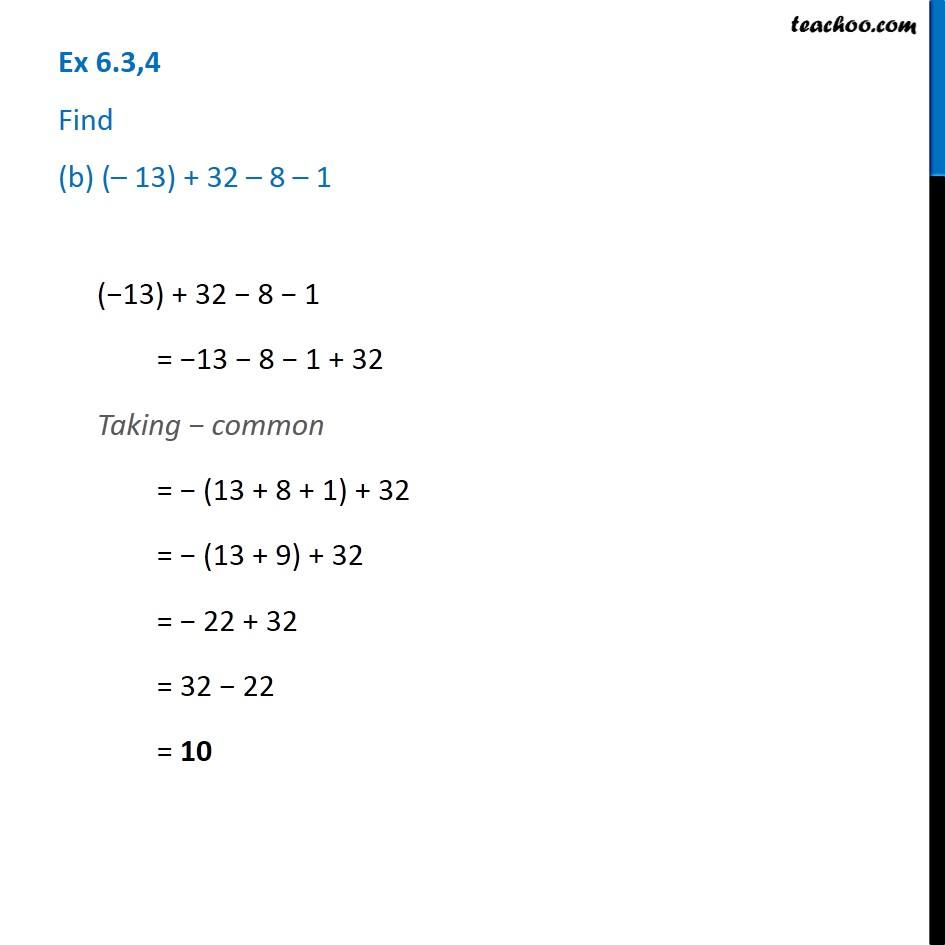1. Chapter 6 Class 6 Integers
2. Serial order wise
3. Ex 6.3

Transcript

Ex 6.3,4 Find (b) (– 13) + 32 – 8 – 1(−13) + 32 − 8 − 1 = −13 − 8 − 1 + 32 Taking − common = − (13 + 8 + 1) + 32 = − (13 + 9) + 32 = − 22 + 32 = 32 − 22 = 10

Ex 6.3

Chapter 6 Class 6 Integers
Serial order wise